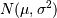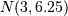# numpy.matlib.randn¶

numpy.matlib.randn(*args)[source]

Return a random matrix with data from the “standard normal” distribution.

randn generates a matrix filled with random floats sampled from a univariate “normal” (Gaussian) distribution of mean 0 and variance 1.

Parameters: *args : Arguments Shape of the output. If given as N integers, each integer specifies the size of one dimension. If given as a tuple, this tuple gives the complete shape. Z : matrix of floats A matrix of floating-point samples drawn from the standard normal distribution.

rand, random.randn

Notes

For random samples from, use:

sigma * np.matlib.randn(...) + mu

Examples

>>> import numpy.matlib
>>> np.matlib.randn(1)
matrix([[-0.09542833]])                                 #random
>>> np.matlib.randn(1, 2, 3)
matrix([[ 0.16198284,  0.0194571 ,  0.18312985],
[-0.7509172 ,  1.61055   ,  0.45298599]])       #random


Two-by-four matrix of samples from:

>>> 2.5 * np.matlib.randn((2, 4)) + 3
matrix([[ 4.74085004,  8.89381862,  4.09042411,  4.83721922],
[ 7.52373709,  5.07933944, -2.64043543,  0.45610557]])  #random


#### Previous topic

numpy.matlib.rand

#### Next topic

Miscellaneous routines